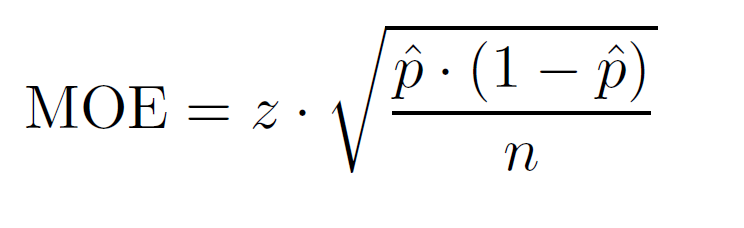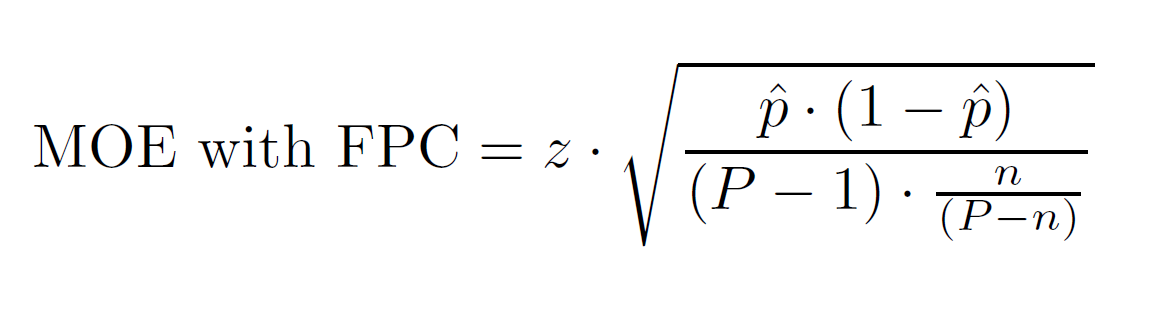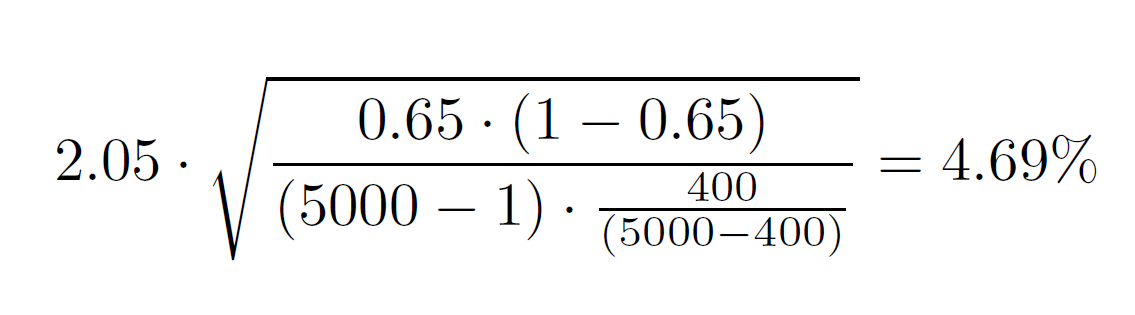# Margin of Error Calculator

Created by Maria Kluziak
Reviewed by Bogna Szyk and Jack Bowater
Last updated: Mar 10, 2020

The margin of error calculator is a useful tool which will allow you to save time when calculating random sampling error in your research. If you:

• need to get the margin of error calculated quickly,
• want to learn how to calculate margin of error,
• are looking for the margin of error formula,
• don't quite know what is a margin of error yet, and you want to read a quick summary to help you grasp the subject,

this calculator is just what you need!

## What is margin of error?

Margin of error is a statistic which states by how many percent the results of a survey may differ from reality. In other words, it's a way of expressing the amount of random sampling errors in a result. It is typically defined as the range of a confidence interval for a particular statistic.

In essence, one can think about it as a way of measuring how effective a survey will be. The smaller the margin of error, the more likely that your results reflect the reality of the entire population, rather than just the sample group. It's an important and useful statistical tool, and understanding its value will help you conduct research in a more precise and confident manner.

## How to use the margin of error calculator?

To use the margin of error calculator, follow these few simple steps:

• Start by deciding whether you want to include the Finite Population Correction factor (FPC) in your calculations. Not sure if it's appropriate? The general rule states that you should account for FPC when the sample size is five or more percent of the entire population. This is important, as the size of the sample impacts the metrics drawn from the data, such as the variance and the standard deviation of the sampling distribution.
• Input the sample size, and, if you're calculating with FPC, the population size.
• Choose the confidence level you are aiming for. The calculator will use this to find the appropriate Z-score.
• The last thing to do is to calculate the sample proportion (known as , or p-hat). To do that, input the number of people who gave the answer you are interested in for your survey. For example, if 100 people took your survey and 57 of them answered "yes" to the question you asked, you would input 57 into this field, and the value of p̂ would be 0.57 (57 / 100).

With all these values, the margin of error calculator will provide you with your MOE in no time.

## How to calculate margin of error?

Now that you know what is margin of error, you might want to expand that knowledge. Sure, our calculator can do the work for you, but we understand that you may feel more confident in your research if you are able to do the calculations on your own.
So how to calculate margin of error? Although it may sound difficult at first, in reality, it's quite a straightforward task. The simple margin of error formula is as follows:Where:

• z stands for the Z-score.
• stands for the sample proportion.
• n stands for the sample size.

The formula for margin of error with Finite Population Correction is as follows:Where:

• z stands for the Z-score.
• stands for the sample proportion.
• n stands for the sample size.
• P stands for the population size.

## Using the margin of error formula - example

For clarity's sake, let's go through an example.

Let's imagine we're surveying 400 people, who represent a population of 5000. After getting the results, we learn that 260 of those people answered "yes" to the survey. As we are quite confident in our research, our confidence level is 96%.

As 400 is 8% of 5000 (feel free to use the percentage calculator to double-check), we have to opt for the margin of error formula which includes FPC.

To sum up, the numbers we are working with are:

• confidence level = 96%, which corresponds to a Z-score of 2.05 (check the next paragraph if you need more information on Z-scores), so:
• z = 2.05
• P = 5000
• n = 400
• p̂ = 260/400 = 0.65

By inputting the above values into the formula, we get the following calculation:The result tells us that our data will be within 4.69 percentage points of the real population value 96% of the time.

## So what about those Z-scores?

You can't really understand how to calculate margin of error if you ignore the concept of Z-score.
In short, Z-score is a numerical statistic that measures the value's relationship to the mean (average) of a group of values, measured in terms of standard deviations from the mean. A Z-score of 0 indicates a value identical to the mean, Z-score of 1 would indicate a value exactly one standard deviation from the mean, and so on and so forth.

What is of interest in this margin of error calculator is which Z-score corresponds to a certain confidence level. A confidence level can be defined as the probability that the value of a parameter falls within a specified range of values.

Different science areas traditionally make use of varying confidence levels. For example, in psychological research, it is most common to opt for a confidence level of 95% (corresponding Z-score of 1.96). In medical research, on the other hand, it is not uncommon to go for higher levels, such as 98% or even 99%. After all, there is not much margin for errors in healing people.

Luckily, the relationship between confidence levels and Z-scores is formalised, and you generally don't need to calculate the Z-score based on a confidence level on your own - there exist widely available resources, such as tables, that tell you precisely what Z-score corresponds to your desired confidence levels. Below you will find an excerpt from such a table, containing some of the confidence levels most widely used in various areas of statistical research.

Z-scores of some of the most common confidence levels:

Confidence level Z-score
70% 1.04
75% 1.15
80% 1.28
85% 1.44
90% 1.645
92% 1.75
95% 1.96
96% 2.05
98% 2.33
99% 2.58
Maria Kluziak
Calculate MOE with Finite Population Correction?
Yes
Data
Confidence level
95%
Sample size
Population size
FPC factor should be included when the sample is at least 5% of the population. Use the percentage calculator to check the relation between sample and population size, and choose "No" in the first field of this calculator if it is less than 5%.
Sample proportion calculation
Number of people who gave the desired answer
Sample proportion (p̂)
Result
Margin of error
%
People also viewed…

### Snowman

The perfect snowman calculator uses math & science rules to help you design the snowman of your dreams!

### Standard deviation

The standard deviation calculator tells you the mean and standard deviation of a dataset.

### Sunbathing

Do you always remember to put on sunscreen before going outside? Are you sure that you use enough? The Sunbathing Calculator ☀ will tell you when's the time to go back under an umbrella not to suffer from a sunburn!

### Z score

The z-score calculator can help you determine the standard score for a data point.